# Honors Algebra 1: Graphing & Factoring Quadratic Equations Chapter Exam

Exam Instructions:

Choose your answers to the questions and click 'Next' to see the next set of questions. You can skip questions if you would like and come back to them later with the yellow "Go To First Skipped Question" button. When you have completed the practice exam, a green submit button will appear. Click it to see your results. Good luck!

### Page 1

#### Question 2 2. Find the solution to the equation below.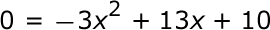### Page 2

#### Question 6 6. Factor and solve.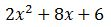#### Question 7 7. How many more women enjoyed watching Alvin and the Chipmunks than men?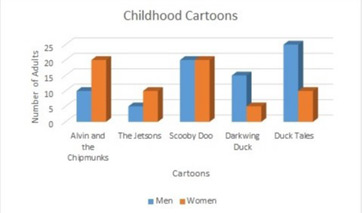#### Question 10 10. Rewrite the following equation in vertex form.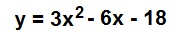### Page 3

#### Question 11 11. Factor.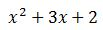### Page 4

#### Question 16 16. In order to complete the square on the following equation, what value would you add to both sides?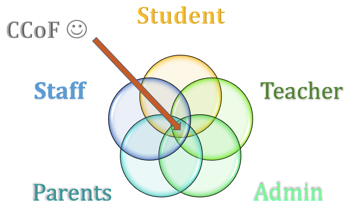#### Question 17 17. Find the value for c that must be added to both sides, and rewrite the equation in the vertex form.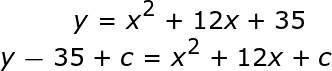#### Question 18 18. Factor and solve.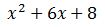#### Question 20 20. What is the interval of the double bar graph?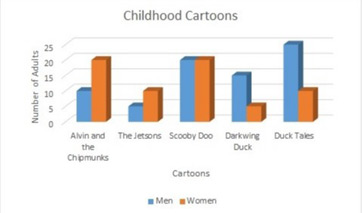### Page 5

#### Question 24 24. What is the total count for Duck Tales?#### Question 25 25. If the equation below told you a ball was 'h' feet in the air after 't' seconds, what would be the first step to finding out when it hit the ground?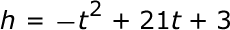### Page 6

#### Question 26 26. Factor and solve.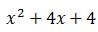#### Question 28 28. Find the solution to the equation below.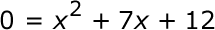#### Question 29 29. Expressing the equation below in the vertex form will give a value of 'h' = _____.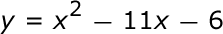#### Honors Algebra 1: Graphing & Factoring Quadratic Equations Chapter Exam Instructions

Choose your answers to the questions and click 'Next' to see the next set of questions. You can skip questions if you would like and come back to them later with the yellow "Go To First Skipped Question" button. When you have completed the practice exam, a green submit button will appear. Click it to see your results. Good luck!

Support